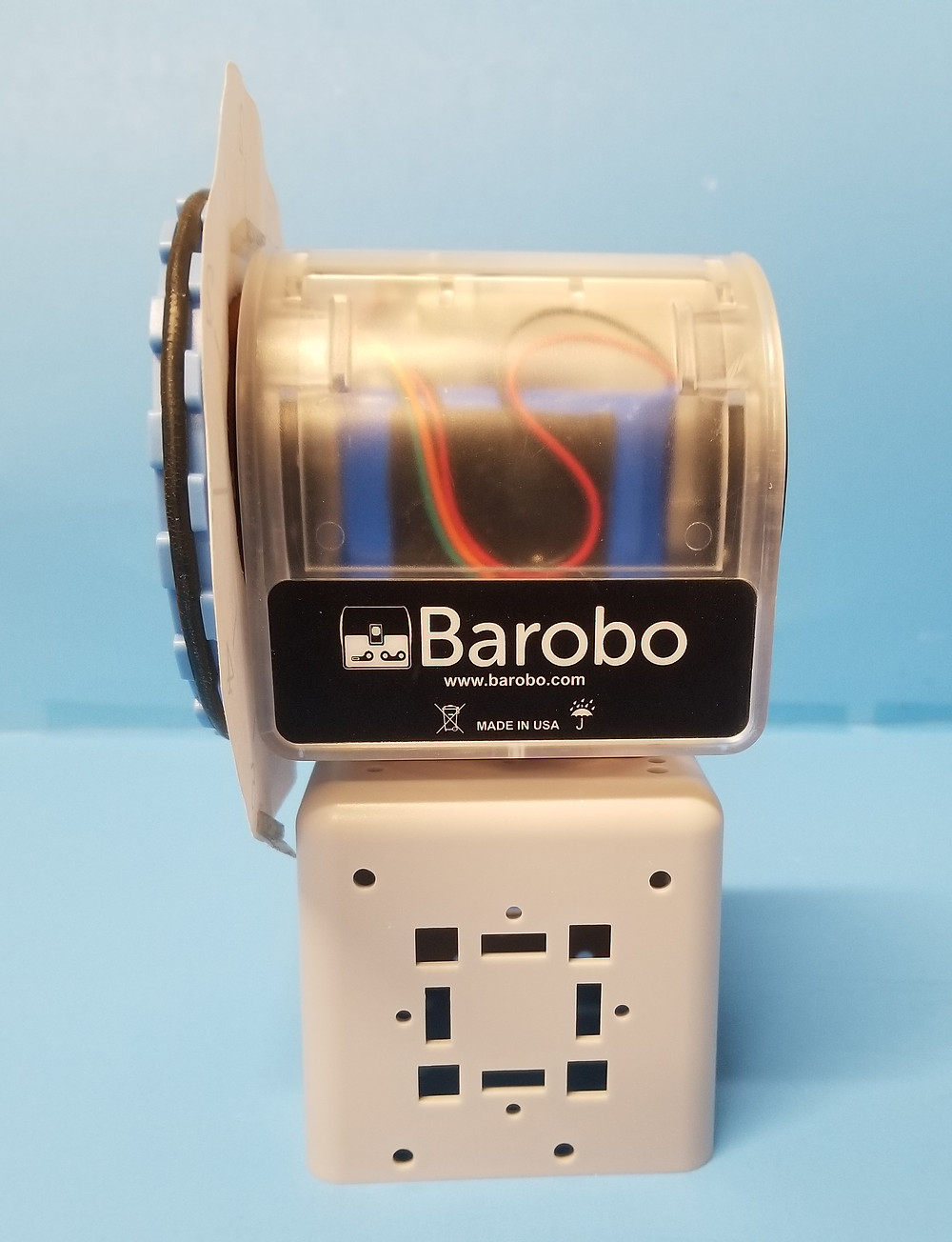# [Project] A Linkbot Clock System

Introduction:

Linkbot is a reconfigurable modular educational robot. In this project, a Clock Linkbot System, consisting of one Linkbot-I, is used as an example to demonstrate how a simple Linkbot coding can help create a clock system. The system can be used to learn the math concepts of angle, clock, ratio, and proportion, etc.

Information:

• Duration: 2-10 Hours

• Level: Beginn

Products Used in the Project:

• Cube Connecto

Parts Used in the Project:

• 1 Linkbot-I (used as the base for the clock)

• 1 Cube Connector (used as a stand)

• 2 Snap Connector

• 1 x 3.5” Wheel

• Clock Print / and cut out​

Tools Used in the Project:

• Scissors

• Tape

Setup:

First, the paper clock handout is reshaped by cutting out the smaller, grey circle with the scissors. Then, cut slightly outside the black outline to leave the rest of the circular clock design. Once the clock is cut out, the Linkbot I is set up by placing the part of the clock that was cut out onto joint 1. Tape the paper clock on the sides of the Linkbot without taping the paper clock on the moving joint itself. The number 12 on the clock should be facing straight upwards while the number 6 should be pointing straight downwards. Once the paper clock is taped accurately, attach the snap connector into joint 1 on the Linkbot I. This ensures that the wheel moves at the same time as the joint. Then, add the wheel on the snap connecter. The wheel should now be on top of the clock and snap connector, as shown in figure one. Next, attach a snap connector to joint 2 (The bottom) of the Linkbot. Attach the cube connecter under this snap connector to create a stand for the clock.Figure 1: The configuration with Linkbot-I parts and the paper clock for the Clock Linkbot System.Figure 2: The clock with the wheel attached and arrow pointed at the timeFigure 3: Back view of clockFigure 4: Side view of clock

Programming the Raspberry Pi Linkbot Siren System in Ch:

A simple Ch program clock1.ch can be used to control this Clock System. Details for each robot member function for CLinkbotI can be found in the textbook “Learning Robot Programming with Linkbot for the Absolute Beginner”. The entire PDF file for this textbook is available in C-STEM Studio.

/* File: clock1.ch

Change the time on a Clock Linkbot system */

double clockTime, angle;

// Move to zero position

robot.resetToZero();

// User inputs the clock time

// -30 is used to convert clock time into angle on linkbot

printf(“Enter the clock time desired.\n”);

scanf(“%lf”, &clockTime);

angle = -30*clockTime;

// Move joint 1 to the clock time wanted

robot.moveJoint(Joint1, angle);

The clock1.ch program first reset the robot’s joints to the zero position with

robot.resetToZero();

Then, the code prompts user input for the hour of the clock and stores the value in the double type variable clockTime:

printf(“Enter the clock time desired.\n”);

scanf(“%lf”, &clockTime);

The angle Joint 1 needs to turn to reach the user-defined time is calculated from:

.

A circular clock has 12 hour-marks and 360o, so each hour is 30o apart. The negative value stands for clockwise turn (math standard set positive angle to be counter-clockwise, but a clock goes clockwise direction)

Finally, the code robot.moveJoint(Joint1, angle); turns Joint1 by the calculated angle, subsequently moving the arrow to the desired time.

A more complex Ch program clock2.ch can be used to control this Clock System as well.

/* File: clock2.ch

Change the time on a Clock Linkbot system */

double clockTime, angle, currentAngle;

int n = 1;

// Move to zero position

robot.resetToZero();

while (n < 4){

// User inputs the clock time

// -30 allows clocktime to be converted into linkbot angle

printf(“Enter the clock time desired.\n”);

scanf(“%lf”, &clockTime);

angle = -30*clockTime;

// currentAngle accounts for whatever time is set on clock

currentAngle = angle – currentAngle;

if (currentAngle > 0)

currentAngle = currentAngle – 360;

// Move joint 1 to the clock time wanted

// currentAngle function sets the user inputted time to the // angle the clock will move to in the next function

robot.moveJoint(Joint1, currentangle);

currentAngle = angle;

n = n + 1;

}

The clock2.ch program is an extension of clock1.ch program; this program allows user to “wind” the clock four times. Joint1 is zeroed and the “winding” action is put inside a counter while loop. Inside the loop, user is prompted to input a time and the angle is calculated as in clock1.ch program.